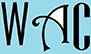## Geometry Question for Title 1 Schools: Straight Line Angle Question?

5) What is the value of an angle of the rest of a straight line that has an angle of 135o ?

The total angle on a straight line  = 180o

If part of the total angle on a line is already 135o ,  then the rest must be 180o  – 135o

= 45o